# SSH模型中的Wilson loop（附Python代码）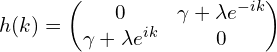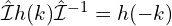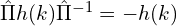SSH模型同时满足以上这两种对称性，因此偶极矩是量子化的。当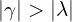时，极化；当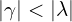时，极化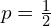"""
This code is supported by the website: https://www.guanjihuan.com
The newest version of this code is on the web page: https://www.guanjihuan.com/archives/10064
"""

import numpy as np
import cmath
from math import *

def hamiltonian(k):  # SSH模型哈密顿量
gamma = 0.5
lambda0 = 1
h = np.zeros((2, 2), dtype=complex)
h[0,0] = 0
h[1,1] = 0
h[0,1] = gamma+lambda0*cmath.exp(-1j*k)
h[1,0] = gamma+lambda0*cmath.exp(1j*k)
return h

def main():
Num_k = 100
k_array = np.linspace(-pi, pi, Num_k)
vector_array = []
for k in k_array:
vector  = get_occupied_bands_vectors(k, hamiltonian)
vector_array.append(vector)
# vector_array.append(vector*cmath.exp(1j*np.random.uniform(0, pi))) # 随机相位测试

# 波函数固定一个规范
vector_sum = 0
for i0 in range(Num_k):
vector_sum += np.abs(vector_array[i0])
index = np.argmax(np.abs(vector_sum))
for i0 in range(Num_k):
vector_array[i0] = find_vector_with_fixed_gauge_by_making_one_component_real(vector_array[i0], index=index)

# # 波函数固定一个规范
# import guan
# vector_array = guan.find_vector_array_with_fixed_gauge_by_making_one_component_real(vector_array, precision=0.005)

# 计算Wilson loop
W_k = 1
for i0 in range(Num_k-1):
F = np.dot(vector_array[i0+1].transpose().conj(), vector_array[i0])
W_k = np.dot(F, W_k)
nu = np.log(W_k)/2/pi/1j
# if np.real(nu) < 0:
#     nu += 1
print('p=', nu, '\n')

def get_occupied_bands_vectors(x, matrix):
matrix0 = matrix(x)
eigenvalue, eigenvector = np.linalg.eig(matrix0)
vector = eigenvector[:, np.argsort(np.real(eigenvalue))]
return vector

def find_vector_with_fixed_gauge_by_making_one_component_real(vector, precision=0.005, index=None):
if index == None:
index = np.argmax(np.abs(vector))
sign_pre = np.sign(np.imag(vector[index]))
for phase in np.arange(0, 2*np.pi, precision):
sign =  np.sign(np.imag(vector[index]*cmath.exp(1j*phase)))
if np.abs(np.imag(vector[index]*cmath.exp(1j*phase))) < 1e-9 or sign == -sign_pre:
break
sign_pre = sign
vector = vector*cmath.exp(1j*phase)
if np.real(vector[index]) < 0:
vector = -vector
return vector

if __name__ == '__main__':
main()


p= (-0.5+0.009257772639491353j)

def hamiltonian(k):  # SSH模型哈密顿量
gamma = 1.5
lambda0 = 1
h = np.zeros((2, 2), dtype=complex)
h[0,0] = 0
h[1,1] = 0
h[0,1] = gamma+lambda0*cmath.exp(-1j*k)
h[1,0] = gamma+lambda0*cmath.exp(1j*k)
return h

p= (-6.308806949364591e-17+0.003169518547424918j)

def hamiltonian(k):
gamma = 0.5
lambda0 = 1
delta = 0.3
h = np.zeros((2, 2), dtype=complex)
h[0,0] = delta
h[1,1] = -delta
h[0,1] = gamma+lambda0*cmath.exp(-1j*k)
h[1,0] = gamma+lambda0*cmath.exp(1j*k)
return h

p= (0.328981519117191+0.007898978069732571j)

"""
This code is supported by the website: https://www.guanjihuan.com
The newest version of this code is on the web page: https://www.guanjihuan.com/archives/10064
"""

import numpy as np
import cmath
from math import *

def hamiltonian(k):
gamma = 0.5
lambda0 = 1
delta = 0
h = np.zeros((2, 2), dtype=complex)
h[0,0] = delta
h[1,1] = -delta
h[0,1] = gamma+lambda0*cmath.exp(-1j*k)
h[1,0] = gamma+lambda0*cmath.exp(1j*k)
return h

def main():
Num_k = 100
k_array = np.linspace(-pi, pi, Num_k)
vector_array = []
for k in k_array:
vector  = get_occupied_bands_vectors(k, hamiltonian)
if k != pi:
vector_array.append(vector)
else:
vector_array.append(vector_array)

# 计算Wilson loop
W_k = 1
for i0 in range(Num_k-1):
F = np.dot(vector_array[i0+1].transpose().conj(), vector_array[i0])
W_k = np.dot(F, W_k)
nu = np.log(W_k)/2/pi/1j
# if np.real(nu) < 0:
#     nu += 1
print('p=', nu, '\n')

def get_occupied_bands_vectors(x, matrix):
matrix0 = matrix(x)
eigenvalue, eigenvector = np.linalg.eig(matrix0)
vector = eigenvector[:, np.argsort(np.real(eigenvalue))]
return vector

if __name__ == '__main__':
main()


 和YZZ同学的讨论

5,826 次浏览

【说明：本站主要是个人的一些笔记和代码分享，内容可能会不定期修改。为了使全网显示的始终是最新版本，这里的文章未经同意请勿转载。引用请注明出处：https://www.guanjihuan.com

## 38 thoughts on “SSH模型中的Wilson loop（附Python代码）”

1.匿名说道：

关老师，为什么我用二分法找相似规范，每次都是找不到规范呢？这是代码，您能帮我看看吗？

import guan
import numpy as np
import cmath
from math import *

def hamiltonian(kx,kw):
kap1 = 0.6
gam1 = 1

kap2 = kap1
gam2 = gam1
d = 1
Ome = 1
h = np.zeros((4,4), dtype=complex)
h[0, 1] = kap2 + gam2*cmath.exp(-1j*kx*d)
h[0, 3] = kap1 + gam1*cmath.exp(-1j*kw*Ome)
h[1, 0] = np.conj(h[0, 1])
h[1, 2] = kap1 + gam1*cmath.exp(-1j*kw*Ome)
h[2, 1] = np.conj(h[1, 2])
h[2, 3] = kap2 + gam2*cmath.exp(1j*kx*d)
h[3, 0] = np.conj(h[0, 3])
h[3, 2] = np.conj(h[2, 3])
return h

Nkx = 101
Nkw = 101
kx_array = np.linspace(-pi, pi, Nkx)
kw_array = np.linspace(-pi, pi, Nkw)
dkx = kx_array - kx_array
dkw = kw_array - kw_array
eig_value_array = np.zeros((Nkx, Nkw, 4),dtype=complex)
px0 = 0

for k0 in range(Nkx-1):
kx = kx_array[k0]
for j0 in range(Nkw-1):
kw = kw_array[j0]

eig_value, eig_vector = np.linalg.eigh(hamiltonian(kx, kw))
vector = eig_vector[:, 0]   # (kx,kw)处的波函数
eig_value_array[k0, j0, :] = eig_value

eig_value, eig_vector = np.linalg.eigh(hamiltonian(kx+dkx, kw))
vector_kx = eig_vector[:, 0]   # 略偏离kx的波函数

rand = np.random.uniform(-pi, pi)
vector_kx = vector_kx * cmath.exp(-1j * rand)

vector_kx = guan.find_vector_with_the_same_gauge_with_binary_search(vector_kx, vector)

Ax = np.dot(vector.transpose().conj(), (vector_kx-vector)/dkx)
px0 = px0 + Ax*dkx*dkw
Px = px0/(2*pi)/(2*pi)


1.guanjihuan说道：

你算的这个波函数的本征值是简并的吧，如果有简并的情况，我目前还不知道怎么处理，因为波函数的任意线性组合都是波函数本身，而且波函数也无法根据本征值进行排序。简并的情况可以参考这篇（目前代码并不是很高效，仅供参考）：非简并波函数和简并波函数的固定规范

2.匿名说道：

若占据态波函数有4个，那么F是不是应该是4*4的矩阵？

1.guanjihuan说道：

这里只考虑一条带，内积后为一个数值

3.匿名说道：

想问问为啥这个用numpy的eigh和eig算出来的数值差异还蛮大的

1.guanjihuan说道：

eig求出的本征值和本征矢量不一定是按本征值的大小排列，需要手动排。而eigh已经做了排列。参考这篇：np.linalg.eig和np.linalg.eigh的区别

4.匿名说道：

感谢博主的分享，想把代码改成matlab学习编程思路。
vector = eigenvector[:, np.argsort(np.real(eigenvalue))] 这句代码的意思是要提取每次计算的特征向量吗？不明白。每次计算都会有两个特征向量。

1.guanjihuan说道：

这个语句表示取价带。因为只有两条带，所以取能量低的一条。

1.匿名说道：

clear
clc
close all

k0=linspace(-pi,pi,100);
for i=1:100
str=zeros(2,1);
k=k0(i);
h1=HH(k);
[V,D]=eig(h1);
vector=V(:,1); %取能量低的一条带
if k~=pi
VV(:,i)=vector;
else
VV(:,i)=V(:,1);
end
end
W_k=1;
for j=1:99
F=dot(VV(:,j+1)',VV(:,j));
ff(:,j)=F;
W_k=dot(F,W_k);
end
nu=log(W_k)/(2*pi*1i);

function h=HH(k)
gamma=0.5;
lambda0=1;
delta=0;
h=zeros(2);
h(1,1)=delta;
h(2,2)=-delta;
h(1,2)=gamma+lambda0*exp(-1i*k);
h(2,1)=gamma+lambda0*exp(1i*k);
end

结果没对，不知道是哪里出了问题
W_k=1.32804668806497e-33 + 5.66626118539546e-49i；
nu=6.79052539801457e-17 + 12.0482836587981i;

1.guanjihuan说道：

在matlab中，dot(A, B)已经包含了对A的厄密共轭， 即：dot(A, B)=A'*B。所以不需要额外再加一撇。

1.匿名说道：

谢谢您

5.匿名说道：

对于计算wilson loop那一块，初始化为1，然后做i0循环。 我想请问 计算下来的F是一个数还是一个矩阵，就涉及到F后面的两个波函数内积的情况，是行矢量点乘列矢量还是列矢量点乘行矢量。 后面是调用np.dot我理解上是矩阵乘法，但是初始化的是一个数。所以我想请问，F是矩阵还是数。 这个问题我可以用博主的代码自己打开看看，先在这里问一下。

1.guanjihuan说道：

内积后是一个数。这里F是一个数，不是矩阵。

6.小志说道：

老师您好，请问对于一些简并能带计算Berry Curvature的时候，需要考虑non-Abelian的Berry Curvature，从定义上虽然能做但会不会波函数的随机相位在数值上会有问题呢？这方面最近有数值方面的工作吗

1.guanjihuan说道：

简并的时候比较麻烦，如果需要波函数连续，可能是要对简并的波函数基做幺正变换。目前我还没做过测试。

7.hmmu说道：

博主您好，请问为什么把本征矢乘以一个，能使模最大分量变实数的一个相因子，就可以达到统一规范的目的呢？（从你的代码中看出来似乎是这个逻辑）

1.guanjihuan说道：

因为波函数乘exp(i*phase)都是方程的解，所以每一个k点的波函数计算可能存在不同的规范。把波函数某个分量变成实数，可以起到固定规范的作用，从而达到波函数连续，这是因为乘一个exp(i*phase)都会导致该分量为非实数。而至于为什么取最大分量，是为了避开波函数分量为0的情况，从而使得代码运行不容易出错。
该方法可以参照文献“An anomalous higher-order topological insulator - An anomalous higher-order topological insulator”的补充材料中的说明。
另外再强调下：Wilson loop的计算可以不考虑波函数的规范任意性（前提是-pi和pi的位置用同一个波函数）。

1.hmmu说道：

这个分量在各个波函数中也不必是同一个位置的是吧？谢谢您的回复和提供的文献，我再学习下这篇文献。

1.hmmu说道：

刚看到下面的回复，原来是要固定index的。

2.guanjihuan说道：

局部使得波函数最大分量变实数可能还不一定使得波函数连续，需要考虑整个布里渊区，目前代码已经完成补充修改。我猜是存在两个k点的最大分量处于不同位置的情况，尤其是临界点附近（即存在两个最大分量），所以需要全程固定一个指标（index），虽然旧代码在这个案例中没出现错误。
但这种方案仍存在一点问题，需要小心存在局部k点该分量为0左右。即使该分量整个布里渊区是最大的，但不排除某些k点为0+或0-。对于这种情况，需要提高相位查找的精度来解决。

8.萌星说道：

请问这个波函数的规范是什么含义呢？
之前计算一个周期晶格的不变量，跟wilson loop非常像，计算过程相同，但是波函数用的是bloch函数的周期部分(即补上相位exp(ikr)以后的部分)，积分路径是k空间上的若干个小平行四边形，结果吻合得很好。中间好像没有涉及到对波函数的规范，所以不太明白规范的具体意义

1.guanjihuan说道：

不变量和可观测量都是和规范的无关的（gauge independent）。波函数相位的选取主要是在数值计算过程中会产生，例如求导时相邻的两个波函数需要连续。这里计算wilson loop也不需要选取规范，前提是把-pi和pi两个点选取为同一个波函数（这两个点本身就是同一个状态）。

1.匿名说道：

明白了，谢谢老师！

9.匿名说道：

作者你知道如何算2D ssh模型的极化数吗？

1.guanjihuan说道：

还没算过。

10.匿名说道：

请问如果有多个价带的话怎么算呢？

1.guanjihuan说道：

如果带不相交或者简并，照样算就可以了。如果存在多个带相交或者简并，会麻烦一些，目前我还没考虑。

11.Kabu Xiong说道：

看到有文章说Wilson loop是规范不变的，但我也没细究
https://doi.org/10.1088/1367-2630/ab3f71

1.guanjihuan说道：

感谢提供的资料。Wilson loop是规范不变的。代码稍微调整下，是不需要对波函数进行规范，博文已经更新。

12.匿名说道：

作者您好！我想请问一下，如何用python循环出SSH模型的实空间中的哈密顿？谢谢！

1.guanjihuan说道：

写出实空间的哈密顿量需要是有限长度。编完号，把每一个跃迁项都赋值下（循环赋值）。参考：方格子模型的哈密顿量形式（在实空间中）

1.匿名说道：

明白了，谢谢您

13.匿名说道：

作者你好，我想问一下，“ # 波函数固定一个规范”那一步是为什么呀，我用你的代码把这一步去掉后，也能得到相同的结果。如果不规范会有什么问题吗

1.guanjihuan说道：

如果把vector_array.append(vector)修改成vector_array.append(vector*cmath.exp(1j*np.random.uniform(0, 1)))，也就是每个波函数加一个随机的相位。这时候只有加了波函数规范的代码，结果才是正确的。

1.匿名说道：

哦哦，明白些了。还有一个问题，就是我想问一下那个波函数规范的公式原型是怎样的呀，我从代码上分析不出来，也没有看到相关的资料说明。谢谢！

1.guanjihuan说道：

目前没看到什么公式，只要使得波函数连续即可。可以自己写代码进行判断。

固定一个规范的目的也是使得波函数连续。这里代码选取的规范是使波函数的最大分量（不为零的分量）的虚部为零。也可以选取其他规范。

1.匿名说道：

好的，谢谢博主的细心解答！！[玫瑰花]

2.guanjihuan说道：

博文更新了。因为wilson loop是gauge invariant，所以适当调整下代码，是可以不需要对波函数进行规范。